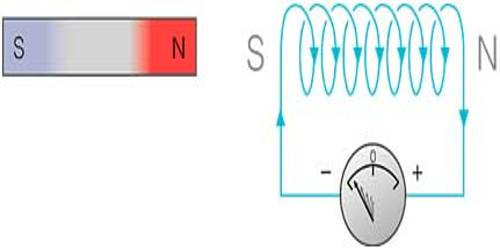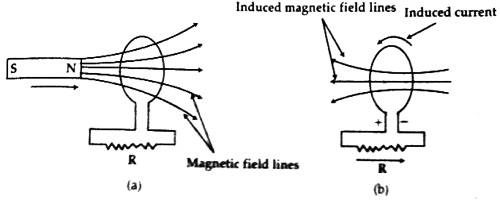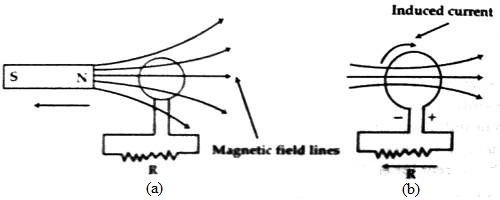Physics

# Explanation of Lenz’s Law of Electromagnetic InductionExplanation of Lenz’s Law of Electromagnetic Induction

Lenz’s Law: In case of electromagnetic induction, the direction of the induced current (or emf) is such as to oppose the change of magnetic flux which causes the production of induced current (or emf) in the circuit.

In other words, the direction of induced current is such as to oppose the very cause which is responsible for inducing the current (or emf) in the circuit.

Explanation: Lenz’s law will be explained by the following example.

(1) Suppose the North Pole N of a bar magnet NS IN approaching to a coil [Fig. (a)]. A resistance R is connected in the external circuit of the coil.As the bar magnet is approaching nearer and nearer to the coil, the magnetic field in the coil will continue to increase. As a result, the magnetic field lines of magnetic flux will also increase. Now according to Lenz’s law, the magnetic field created due to the induced current in the coil will oppose the change in magnetic flux. In order to oppose the change in magnetic flux the direction of the induced magnetic field must be opposite to the magnetic field of the bar magnet. In the figure since the field lines of the bar magnet are passing through the coil from left to right, so magnetic field lines due to the induced current will pass from right to left through the coil [fig (b)]. For this type of induced field, the direction of induced current in the coil must be (according to the right-hand rule) counterclockwise. The coil will act as a source of electromotive force like a battery. Positive and negative terminals of the electromotive force will be similar to that shown in the figure.

If the bar magnet recedes from the coil the reverse situation will occur. That means the magnetic field lines or flux through the coil will decrease [Fig. 2(a)]. According toLenz’s law the direction of the magnetic field due to the induced current in the coil will be such as to oppose the decrease of flux. In this case, the direction of current will be clockwise [Fig. 2(b)]. So, combining both the Faraday’s and Lenz’s laws we get,

E = – φ/t

or, E = – dφ/dt

Here negative sign indicates that E and φ/t or (dφ/dt) act opposite to each other.